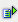The function log10 (num) calculates the logarithm of a value on the basis 10.

Syntax: =log10(num)Example =log10(1) is 0 =log10(10) is 1 =log10(100) is 2 =log10(1000) is 3